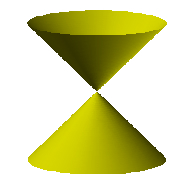# double cone

Double Cone

A geometric figure made up of two right circular cones placed apex to apex as shown below. Typically a double cone is considered to extend infinitely far in both directions, especially when working with conic sections and degenerate conic sections.

Note: The graph of the equationz2 = x2 + y2 is a standard way to represent a double cone. That is the equation for the image below.Double Cone